# Fisseha Berhane, PhD

#### Data Scientist443-970-2353[email protected] CV Resume## Supervised Machine Learning with R and Python¶

Here I show how to build various machine learning models in Python and R. The models include linear regression, logistic regression, tree-based models (bagging and random forest) and support vector machines (SVM).

The data set used for the linear regression models is from here.

### Linear Regression with R¶

In :
setwd("C:/Fish/Python/Python_vs_R")
options(jupyter.plot_mimetypes = 'image/png')
options(repr.plot.width = 6)
options(repr.plot.height = 4)

In :
clim<-read.csv("climate_change.csv")

In :
names(clim)

Out:
1. "Year"
2. "Month"
3. "MEI"
4. "CO2"
5. "CH4"
6. "N2O"
7. "CFC.11"
8. "CFC.12"
9. "TSI"
10. "Aerosols"
11. "Temp"
In :
dim(clim)

Out:
1. 308
2. 11

Let's use the data up to 2006 as training data and test for the years after 2006.

In :
training<-subset(clim,clim$Year<=2006) testing<-subset(clim,clim$Year> 2006)

In :
model1<-lm(Temp~MEI+CO2+CH4+N2O+CFC.11+CFC.12+TSI+Aerosols,data=training)

In :
summary(model1)

Out:
Call:
lm(formula = Temp ~ MEI + CO2 + CH4 + N2O + CFC.11 + CFC.12 +
TSI + Aerosols, data = training)

Residuals:
Min       1Q   Median       3Q      Max
-0.25888 -0.05913 -0.00082  0.05649  0.32433

Coefficients:
Estimate Std. Error t value Pr(>|t|)
(Intercept) -1.246e+02  1.989e+01  -6.265 1.43e-09 ***
MEI          6.421e-02  6.470e-03   9.923  < 2e-16 ***
CO2          6.457e-03  2.285e-03   2.826  0.00505 **
CH4          1.240e-04  5.158e-04   0.240  0.81015
N2O         -1.653e-02  8.565e-03  -1.930  0.05467 .
CFC.11      -6.631e-03  1.626e-03  -4.078 5.96e-05 ***
CFC.12       3.808e-03  1.014e-03   3.757  0.00021 ***
TSI          9.314e-02  1.475e-02   6.313 1.10e-09 ***
Aerosols    -1.538e+00  2.133e-01  -7.210 5.41e-12 ***
---
Signif. codes:  0 '***' 0.001 '**' 0.01 '*' 0.05 '.' 0.1 ' ' 1

Residual standard error: 0.09171 on 275 degrees of freedom
Multiple R-squared:  0.7509,	Adjusted R-squared:  0.7436
F-statistic: 103.6 on 8 and 275 DF,  p-value: < 2.2e-16


In this preliminary model, we see that multiple R-squared is 0.7509 and we all covariates are significant except CH4.

In :
options(repr.plot.width = 6)
options(repr.plot.height = 4)

pred_train<-predict(model1,data=training)

plot(training$Temp,pred_train,col='darkblue',xlab='Observed temperature',ylab='fitted values',pch=16)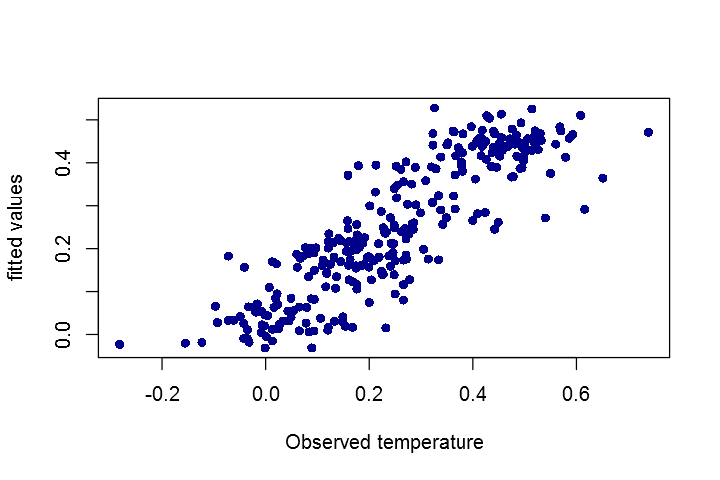In : pred_test<-predict(model1, newdata=testing) plot(testing$Temp,pred_test,col='green',pch=16,xlab='Observed temperature',ylab='Predictions')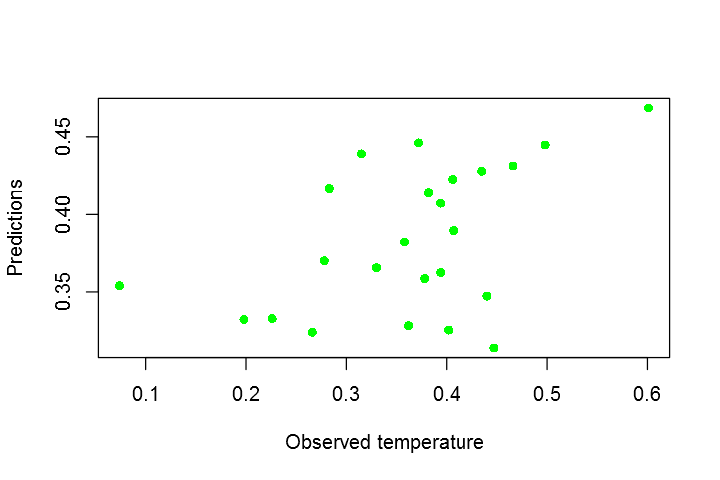### Linear Regression with Python¶

Now, let's see how to build a linear regression model in Python.

In :
import pandas as pd
import numpy as np
import seaborn as sns

import matplotlib.pyplot as plt
%matplotlib inline

from sklearn import metrics
from sklearn.cross_validation import train_test_split


#### Read data into a dataframe using pandas.¶

In :
clim = pd.read_csv(r'https://courses.edx.org/asset-v1:[email protected]+block/climate_change.csv')


Let's look at the covariates.

In :
clim.head(3)

Out:
Year Month MEI CO2 CH4 N2O CFC-11 CFC-12 TSI Aerosols Temp
0 1983 5 2.556 345.96 1638.59 303.677 191.324 350.113 1366.1024 0.0863 0.109
1 1983 6 2.167 345.52 1633.71 303.746 192.057 351.848 1366.1208 0.0794 0.118
2 1983 7 1.741 344.15 1633.22 303.795 192.818 353.725 1366.2850 0.0731 0.137
In :
clim.shape

Out:
(308, 11)

we can also plot the data. Let's visualize the relationship between the features and the response (temperature) using scatterplots with seaborn.

In :
sns.pairplot(clim, x_vars=['MEI','CO2','CH4','N2O'], y_vars='Temp', size=2.5, aspect=1, kind='reg')

Out:
<seaborn.axisgrid.PairGrid at 0x2e07b320>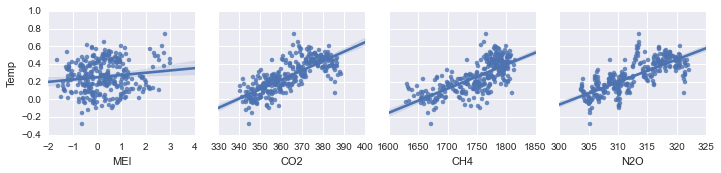In :
sns.pairplot(clim, x_vars=['CFC-11','CFC-12','TSI','Aerosols'], y_vars='Temp', size=2.5, aspect=1, kind='reg')

Out:
<seaborn.axisgrid.PairGrid at 0x1f5997b8>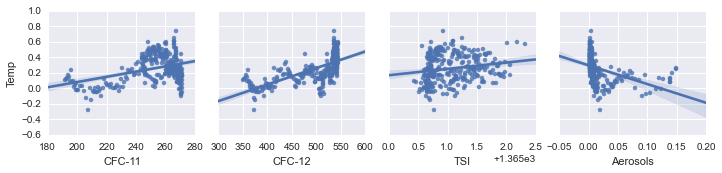We can also plot the scatter plots using pandas.

In :
pd.scatter_matrix(clim[['Year','MEI','CO2','CH4','N2O','CFC-11','CFC-12','TSI','Aerosols','Temp']], figsize=(15, 15),alpha=0.2,diagonal='kde')

plt.show()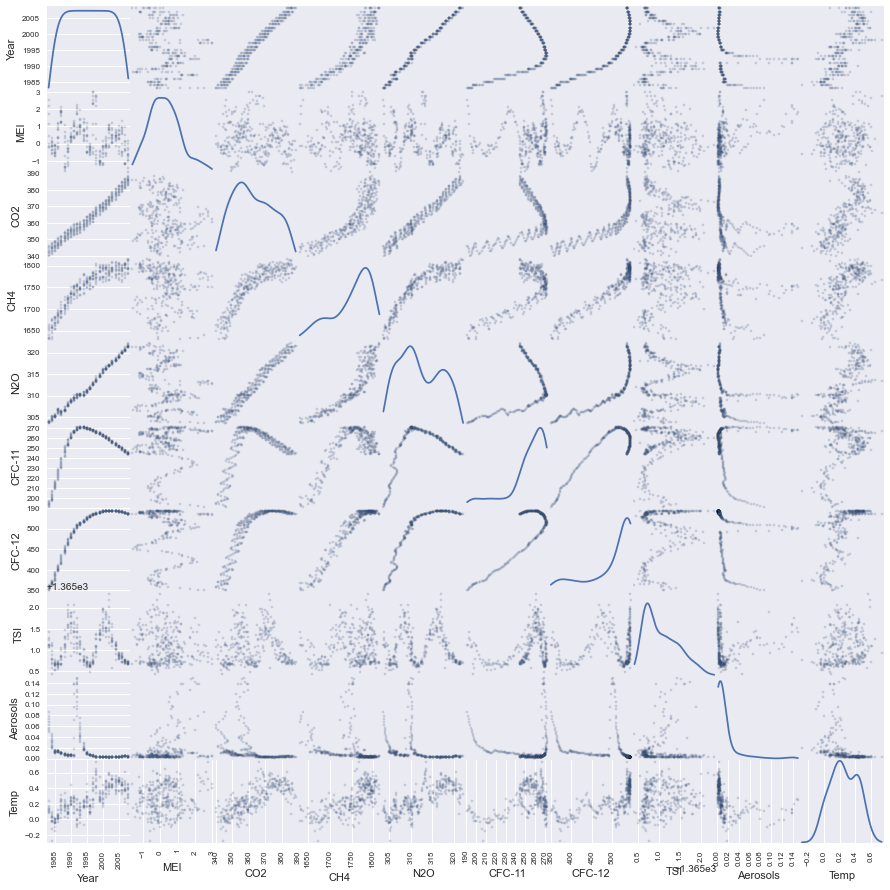In :
training=clim[clim['Year']<=2006]
testing=clim[clim['Year']>2006]

In :
training.shape, testing.shape

Out:
((284, 11), (24, 11))
In :
np.unique(training['Year'])

Out:
array([1983, 1984, 1985, 1986, 1987, 1988, 1989, 1990, 1991, 1992, 1993,
1994, 1995, 1996, 1997, 1998, 1999, 2000, 2001, 2002, 2003, 2004,
2005, 2006], dtype=int64)

The training period covers from 1983 to 2006.

In :
np.unique(testing['Year'])

Out:
array([2007, 2008], dtype=int64)

The testing data covers 2007 and 2008.

In :
### SCIKIT-LEARN ###

from sklearn import linear_model

linear = linear_model.LinearRegression()

# create X and y

feature_cols = ['MEI','CO2','CH4','N2O','CFC-11','CFC-12','TSI','Aerosols']
X = training[feature_cols]
y = training.Temp

#  fit
linear.fit(X, y)

print 'R-squared: %0.2f'%linear.score(X,y)

R-squared: 0.75


or we can calculate the R-squared value using the metrics.r2_score method.

In :
y_pred = linear.predict(X)
round(metrics.r2_score(y, y_pred),2)

Out:
0.75

The R-squared value is 0.75, the same with what R gives us.

print the coefficients

In :
print('Coefficient: \n', linear.coef_)
print('Intercept: \n', linear.intercept_)

('Coefficient: \n', array([  6.42053134e-02,   6.45735927e-03,   1.24041896e-04,
-1.65280033e-02,  -6.63048889e-03,   3.80810324e-03,
9.31410835e-02,  -1.53761324e+00]))
('Intercept: \n', -124.5942604011142)

In :
feature_cols = ['MEI','CO2','CH4','N2O','CFC-11','CFC-12','TSI','Aerosols']
X_test = testing[feature_cols]
predicted= linear.predict(X_test)
obs_pred=pd.DataFrame({"Observation":testing.Temp,"Predicted":predicted})

In :
obs_pred.plot(kind='scatter', x='Observation', y='Predicted',color='DarkBlue')
plt.show()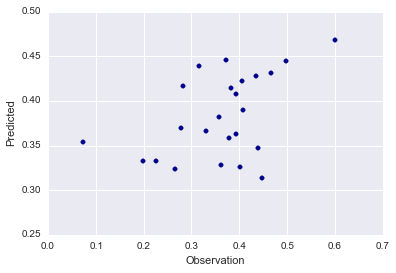or using seaborn:

In :
sns.pairplot(obs_pred, x_vars=['Observation'], y_vars='Predicted', size=10, aspect=1, kind='reg')
plt.show()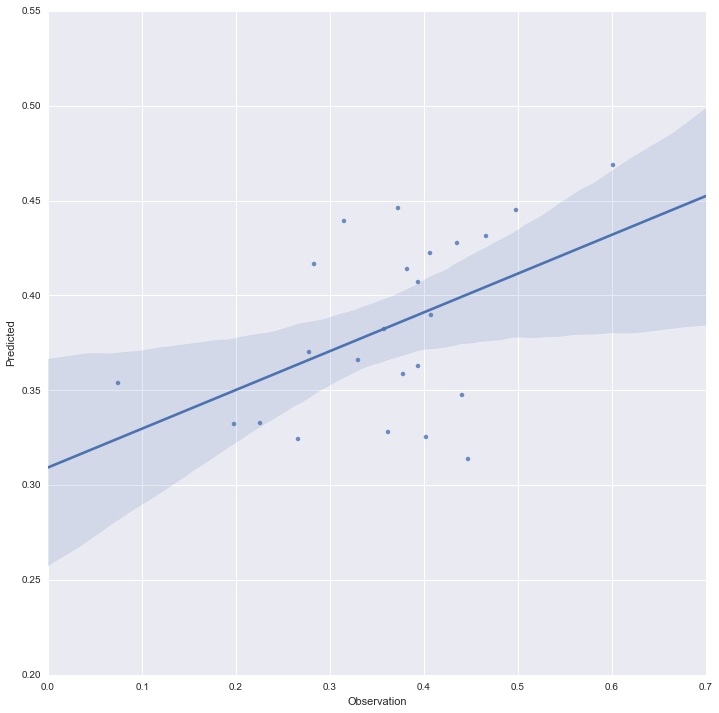### Predicting loan repayment with Logistic Regression¶

Let's predict the risk of a borrower being unable to repay a loan. The data set used here is from LendingClub.com.

Our response is the 'not_fully_paid' variable which shows that loan was not paid back in full. The data used here can be downloaded from here.

### Logistic Regression with R¶

In :
setwd("C:/Fish/Python/Python_vs_R")

In :
loans<-read.csv("loans_imputed.csv")


Let's explore the dataset.

In :
str(loans)

'data.frame':	9578 obs. of  14 variables:
$credit.policy : int 1 1 1 1 1 1 1 1 1 1 ...$ purpose          : Factor w/ 7 levels "all_other","credit_card",..: 3 2 3 3 2 2 3 1 5 3 ...
$int.rate : num 0.119 0.107 0.136 0.101 0.143 ...$ installment      : num  829 228 367 162 103 ...
$log.annual.inc : num 11.4 11.1 10.4 11.4 11.3 ...$ dti              : num  19.5 14.3 11.6 8.1 15 ...
$fico : int 737 707 682 712 667 727 667 722 682 707 ...$ days.with.cr.line: num  5640 2760 4710 2700 4066 ...
$revol.bal : int 28854 33623 3511 33667 4740 50807 3839 24220 69909 5630 ...$ revol.util       : num  52.1 76.7 25.6 73.2 39.5 51 76.8 68.6 51.1 23 ...
$inq.last.6mths : int 0 0 1 1 0 0 0 0 1 1 ...$ delinq.2yrs      : int  0 0 0 0 1 0 0 0 0 0 ...
$pub.rec : int 0 0 0 0 0 0 1 0 0 0 ...$ not.fully.paid   : int  0 0 0 0 0 0 1 1 0 0 ...

In :
set.seed(144)

library(caTools)

split=sample.split(loans$not.fully.paid,SplitRatio = 0.7) train<-loans[split==TRUE, ] test<-loans[split==FALSE, ] mod1<-glm(not.fully.paid~., data=train, family=binomial)  Let's see the significant features. In : summary(mod1)  Out: Call: glm(formula = not.fully.paid ~ ., family = binomial, data = train) Deviance Residuals: Min 1Q Median 3Q Max -2.2049 -0.6205 -0.4951 -0.3606 2.6397 Coefficients: Estimate Std. Error z value Pr(>|z|) (Intercept) 9.187e+00 1.554e+00 5.910 3.42e-09 *** credit.policy -3.368e-01 1.011e-01 -3.332 0.000861 *** purposecredit_card -6.141e-01 1.344e-01 -4.568 4.93e-06 *** purposedebt_consolidation -3.212e-01 9.183e-02 -3.498 0.000469 *** purposeeducational 1.347e-01 1.753e-01 0.768 0.442201 purposehome_improvement 1.727e-01 1.480e-01 1.167 0.243135 purposemajor_purchase -4.830e-01 2.009e-01 -2.404 0.016203 * purposesmall_business 4.120e-01 1.419e-01 2.905 0.003678 ** int.rate 6.110e-01 2.085e+00 0.293 0.769446 installment 1.275e-03 2.092e-04 6.093 1.11e-09 *** log.annual.inc -4.337e-01 7.148e-02 -6.067 1.30e-09 *** dti 4.638e-03 5.502e-03 0.843 0.399288 fico -9.317e-03 1.710e-03 -5.448 5.08e-08 *** days.with.cr.line 2.371e-06 1.588e-05 0.149 0.881343 revol.bal 3.085e-06 1.168e-06 2.641 0.008273 ** revol.util 1.839e-03 1.535e-03 1.199 0.230722 inq.last.6mths 8.437e-02 1.600e-02 5.275 1.33e-07 *** delinq.2yrs -8.320e-02 6.561e-02 -1.268 0.204762 pub.rec 3.300e-01 1.139e-01 2.898 0.003756 ** --- Signif. codes: 0 '***' 0.001 '**' 0.01 '*' 0.05 '.' 0.1 ' ' 1 (Dispersion parameter for binomial family taken to be 1) Null deviance: 5896.6 on 6704 degrees of freedom Residual deviance: 5485.2 on 6686 degrees of freedom AIC: 5523.2 Number of Fisher Scoring iterations: 5  Now, we can predict using the test data. In : predicted.risk=predict(mod1,newdata=test, type="response") test$predicted.risk=predicted.risk


Let's plot the Receiver operating characteristic (ROC) curve.

In :
# load ROCR package

library(ROCR)

In :
# Prediction function

ROCRpred = prediction(test$predicted.risk,test$not.fully.paid)

# Performance function

ROCRperf = performance(ROCRpred, "tpr", "fpr")

# Plot ROC curve

options(repr.plot.width = 8)
options(repr.plot.height = 5)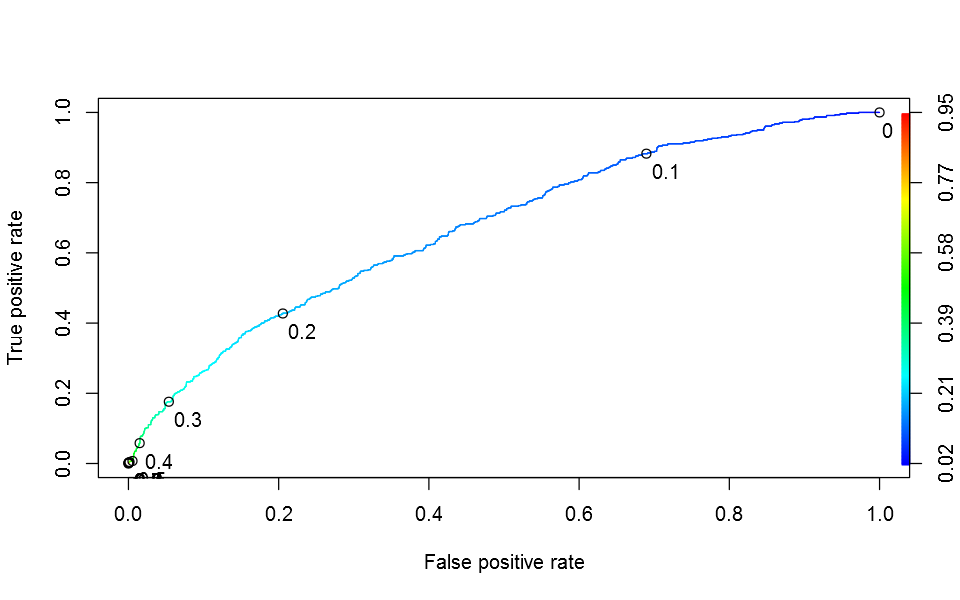Now, let's calculate the area under the curve (AUC) value. AUC is equal to the probability that a classifier will rank a randomly chosen positive instance higher than a randomly chosen negative one (assuming 'positive' ranks higher than 'negative')

In :
# AUC
round(as.numeric(performance(ROCRpred, "auc")@y.values),2)

Out:
0.67

We can also calculate confusion matrix and derivates from the confusion matrix.

In :
# The confusion matrix can be computed with the following commands:

test$predicted.risk = predict(mod1, newdata=test, type="response") table(test$not.fully.paid, test$predicted.risk > 0.5) # using 0.5 as a threshold  Out:  FALSE TRUE 0 2400 13 1 457 3 Now, let's calculate accuracy, sensitivity and specificity. In : accuracy=round(sum(diag(table(test$not.fully.paid, test$predicted.risk > 0.5)))/sum(table(test$not.fully.paid, test$predicted.risk > 0.5)),2) sensitivity=round(3/16,2) specificity=round(2400/(2400+457),2) cat("\tAccuracy = ",accuracy ,"\n", "\tSensitivity = ",sensitivity, "\n","\tSpecificity = ",specificity ,"\n")   Accuracy = 0.84 Sensitivity = 0.19 Specificity = 0.84  ### Logistic Regression with Python¶ In : loan = pd.read_csv(r'http://courses.edx.org/asset-v1:MITx+15.071x_2a+2T2015+type@asset+block/loans_imputed.csv')  Let's have a look at the dataset. In : loan.head(3)  Out: credit.policy purpose int.rate installment log.annual.inc dti fico days.with.cr.line revol.bal revol.util inq.last.6mths delinq.2yrs pub.rec not.fully.paid 0 1 debt_consolidation 0.1189 829.10 11.350407 19.48 737 5639.958333 28854 52.1 0 0 0 0 1 1 credit_card 0.1071 228.22 11.082143 14.29 707 2760.000000 33623 76.7 0 0 0 0 2 1 debt_consolidation 0.1357 366.86 10.373491 11.63 682 4710.000000 3511 25.6 1 0 0 0 In : loan.shape  Out: (9578, 14) Let's create a Python list of feature names and use the list to select a subset of the original DataFrame. In : # create a Python list of feature names feature_cols = ['credit.policy', 'int.rate','installment','log.annual.inc','dti','fico','days.with.cr.line','revol.bal','revol.util' ,'inq.last.6mths','delinq.2yrs','pub.rec'] # use the list to select a subset of the original DataFrame X = loan[feature_cols] # print the first 5 rows of the features. X.head()  Out: credit.policy int.rate installment log.annual.inc dti fico days.with.cr.line revol.bal revol.util inq.last.6mths delinq.2yrs pub.rec 0 1 0.1189 829.10 11.350407 19.48 737 5639.958333 28854 52.1 0 0 0 1 1 0.1071 228.22 11.082143 14.29 707 2760.000000 33623 76.7 0 0 0 2 1 0.1357 366.86 10.373491 11.63 682 4710.000000 3511 25.6 1 0 0 3 1 0.1008 162.34 11.350407 8.10 712 2699.958333 33667 73.2 1 0 0 4 1 0.1426 102.92 11.299732 14.97 667 4066.000000 4740 39.5 0 1 0 Then, let's prepare the dependent variable (response). In : y = loan['not.fully.paid'] # print the first 5 values of the predictand y.head()  Out: 0 0 1 0 2 0 3 0 4 0 Name: not.fully.paid, dtype: int64 In : #Import Library from sklearn.linear_model import LogisticRegression # Create logistic regression object model = LogisticRegression()  Train the model using the training sets and check score #### Splitting X and y into training and testing sets¶ In : from sklearn.cross_validation import train_test_split X_train, X_test, y_train, y_test = train_test_split(X, y, random_state=144,test_size=0.3) # default split is 75% for training and 25% for testing  Check that the training data is 70% of the original data and the rest 30% is allocated for testing. In : print X_train.shape print y_train.shape print X_test.shape print y_test.shape  (6704, 12) (6704L,) (2874, 12) (2874L,)  In : # Create logistic regression object model = LogisticRegression() # Train the model using the training sets and check score model.fit(X_train, y_train) print 'The accuracy of this model is %0.2f' %round(model.score(X_train, y_train),2)  The accuracy of this model is 0.84  The accury is similar using Python and R. In : # Equation coefficient and Intercept print('Coefficient: \n', model.coef_) print('Intercept: \n', model.intercept_) #Predict Output predicted= model.predict(X_test)  ('Coefficient: \n', array([[ -2.92864792e-02, 1.91021226e-03, 8.45693935e-04, 2.47300223e-03, 3.05574553e-03, -3.29998968e-03, -4.21654188e-05, 1.92812967e-06, 5.57896478e-03, 1.36024983e-01, 2.14731566e-03, 7.73942901e-03]])) ('Intercept: \n', array([ 0.00289314]))  ## Decison trees with R¶ Let's predict the risk of a borrower being unable to repay a loan. The data set used here is from LendingClub.com. Our response is the 'not_fully_paid' variable which shows that loan was not paid back in full. The data used here can be downloaded from here. In : setwd("C:/Fish/Python/Python_vs_R")  In : library(caTools) loans<-read.csv("loans_imputed.csv") set.seed(144) library(caTools) split=sample.split(loans$not.fully.paid,SplitRatio = 0.7)

train<-loans[split==TRUE, ]
test<-loans[split==FALSE, ]

In :
library(rpart)

library(rpart.plot)

In :
CARTmodel = rpart(not.fully.paid~., data=train, method="class",cp=0.002)

prp(CARTmodel)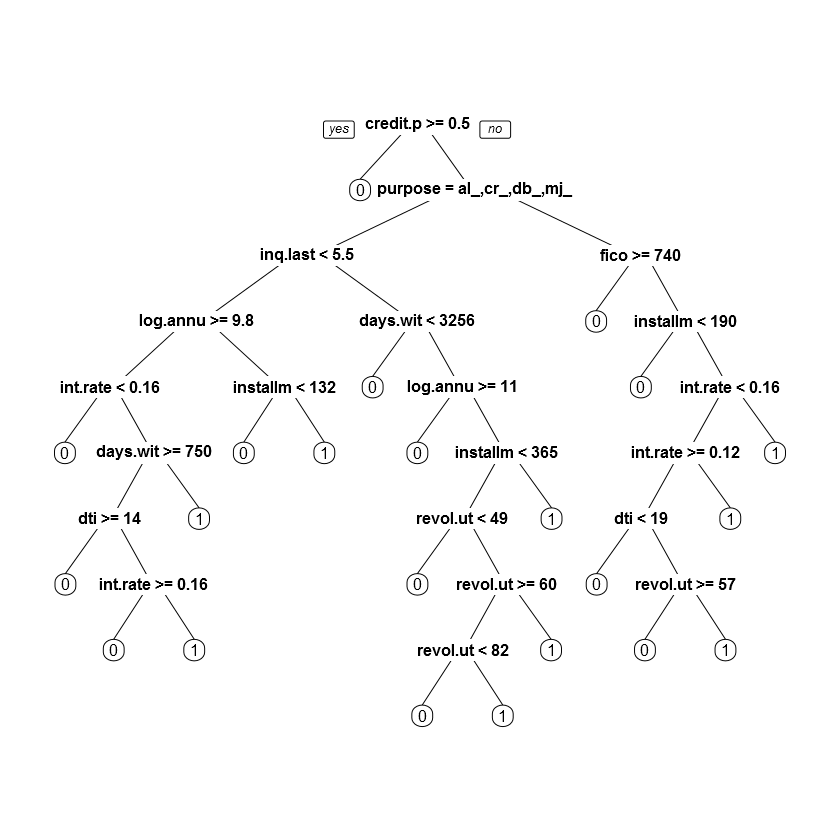In :
prediction<-predict(CARTmodel, type="class",newdata=test)

table<-table(prediction,test$not.fully.paid) accuracy<-sum(diag(table))/(sum(table)) cat("The accuracy is ",round(accuracy,2))  The accuracy is 0.84 The accuracy is similar to what we found using logistic regression using R and Python. ## Decison trees with Python¶ In : loan = pd.read_csv(r'http://courses.edx.org/asset-v1:MITx+15.071x_2a+2T2015+type@asset+block/loans_imputed.csv')  In : # create a Python list of feature names feature_cols = ['credit.policy', 'int.rate','installment','log.annual.inc','dti','fico','days.with.cr.line','revol.bal','revol.util' ,'inq.last.6mths','delinq.2yrs','pub.rec'] # use the list to select a subset of the original DataFrame X = loan[feature_cols] # print the first 5 rows X.head()  Out: credit.policy int.rate installment log.annual.inc dti fico days.with.cr.line revol.bal revol.util inq.last.6mths delinq.2yrs pub.rec 0 1 0.1189 829.10 11.350407 19.48 737 5639.958333 28854 52.1 0 0 0 1 1 0.1071 228.22 11.082143 14.29 707 2760.000000 33623 76.7 0 0 0 2 1 0.1357 366.86 10.373491 11.63 682 4710.000000 3511 25.6 1 0 0 3 1 0.1008 162.34 11.350407 8.10 712 2699.958333 33667 73.2 1 0 0 4 1 0.1426 102.92 11.299732 14.97 667 4066.000000 4740 39.5 0 1 0 In : # select a Series from the DataFrame y = loan['not.fully.paid'] # print the first 5 values y.head()  Out: 0 0 1 0 2 0 3 0 4 0 Name: not.fully.paid, dtype: int64 In : from sklearn.cross_validation import train_test_split X_train, X_test, y_train, y_test = train_test_split(X, y, random_state=144,test_size=0.3)  In : from sklearn import tree # Create tree object model = tree.DecisionTreeClassifier(criterion='gini') # for classification, here you can change the algorithm as gini or entropy (information gain) by default it is gini # model = tree.DecisionTreeRegressor() for regression # Train the model using the training sets and check score model.fit(X_train, y_train)  Out: DecisionTreeClassifier(class_weight=None, criterion='gini', max_depth=None, max_features=None, max_leaf_nodes=None, min_samples_leaf=1, min_samples_split=2, min_weight_fraction_leaf=0.0, random_state=None, splitter='best') In : from sklearn import tree from sklearn.metrics import accuracy_score clf = tree.DecisionTreeClassifier(min_samples_split=60) # default value of min_samples_split is 2 print round(accuracy_score(clf.fit(X_train,y_train).predict(X_test),y_test),2)  0.82  ### Support Vector Machine (SVM) with R¶ Again, let's predict the risk of a borrower being unable to repay a loan. The data set used here is from LendingClub.com. Our response is the 'not_fully_paid' variable which shows that loan was not paid back in full. The data used here can be downloaded from here. In : setwd("C:/Fish/Python/Python_vs_R")  In : library(caTools) loans<-read.csv("loans_imputed.csv") set.seed(144) library(caTools) split=sample.split(loans$not.fully.paid,SplitRatio = 0.7)

train<-loans[split==TRUE, ]
test<-loans[split==FALSE, ]

In :
library(e1071)

In :
fit = svm(not.fully.paid~., data=train)

In :
summary(fit)

Out:
Call:
svm(formula = not.fully.paid ~ ., data = train)

Parameters:
SVM-Type:  eps-regression
cost:  1
gamma:  0.05263158
epsilon:  0.1

Number of Support Vectors:  2867



Now, let's predict using the test data

In :
predicted= predict(fit,test)


We can calculate the confusion matrix

In :
table(test$not.fully.paid, predicted>0.5)  Out:  FALSE TRUE 0 2412 1 1 459 1 In : cat('Accuracy:=',sum(diag(table(test$not.fully.paid,  predicted>0.5)))/sum(table(test$not.fully.paid, predicted>0.5)))  Accuracy:= 0.8398886 ### Support Vector Machine (SVM) with Python¶ In : loan = pd.read_csv(r'http://courses.edx.org/asset-v1:MITx+15.071x_2a+2T2015+type@asset+block/loans_imputed.csv') # create a Python list of feature names feature_cols = ['credit.policy', 'int.rate','installment','log.annual.inc','dti','fico','days.with.cr.line','revol.bal','revol.util' ,'inq.last.6mths','delinq.2yrs','pub.rec'] X = loan[feature_cols] y = loan['not.fully.paid']  In : from sklearn.cross_validation import train_test_split X_train, X_test, y_train, y_test = train_test_split(X, y, random_state=144,test_size=0.3)  In : #Import Library from sklearn import svm model = svm.SVC(kernel='rbf',C=10000.0) # check with different kernels and C model.fit(X, y) model.score(X, y) #Predict Output predicted= model.predict(X_test)  Calculate accuracy. In : from sklearn.metrics import accuracy_score print 'Accuracy is ', round(accuracy_score(model.fit(X_train,y_train).predict(X_test),y_test),2)  Accuracy is 0.85  ### Random Forest with R¶ In : setwd("C:/Fish/Python/Python_vs_R")  In : library(caTools) loans<-read.csv("loans_imputed.csv") set.seed(144) library(caTools) split=sample.split(loans$not.fully.paid,SplitRatio = 0.7)

train<-loans[split==TRUE, ]
test<-loans[split==FALSE, ]

In :
library(randomForest)

In :
fit = randomForest(as.factor(not.fully.paid)~., data=train)

In :
fit

Out:
Call:
randomForest(formula = as.factor(not.fully.paid) ~ ., data = train)
Type of random forest: classification
Number of trees: 500
No. of variables tried at each split: 3

OOB estimate of  error rate: 16.09%
Confusion matrix:
0  1 class.error
0 5606 26 0.004616477
1 1053 20 0.981360671

Let's see variable importance.

In :
importance (fit)

Out:
MeanDecreaseGini
credit.policy28.60945
purpose90.82407
int.rate187.8175
installment203.7339
log.annual.inc198.4494
dti200.5874
fico138.7061
days.with.cr.line204.8603
revol.bal197.2738
revol.util200.1005
inq.last.6mths98.60741
delinq.2yrs26.74009
pub.rec17.31238

We can plot variable importance from the Random Forest model.

In :
options(repr.plot.width = 8)
options(repr.plot.height = 5)

varImpPlot(fit,main ="Variable Importance of features")In :
prediction=predict(fit, test)

In :
accuracy = sum(prediction==test$not.fully.paid)/length(test$not.fully.paid)
round(accuracy,2)

Out:
0.84

### Random Forest with Python¶

In :
loan = pd.read_csv(r'http://courses.edx.org/asset-v1:MITx+15.071x_2a+2T2015+type@asset+block/loans_imputed.csv')

# create a Python list of feature names

feature_cols = ['credit.policy', 'int.rate','installment','log.annual.inc','dti','fico','days.with.cr.line','revol.bal','revol.util'
,'inq.last.6mths','delinq.2yrs','pub.rec']

X = loan[feature_cols]

y = loan['not.fully.paid']

In :
from sklearn.cross_validation import train_test_split
X_train, X_test, y_train, y_test = train_test_split(X, y, random_state=144,test_size=0.3)

In :
#Import Library

from sklearn.ensemble import RandomForestClassifier

model = RandomForestClassifier()

model.fit(X, y)

model.score(X, y)
#Predict Output
predicted= model.predict(X_test)

In :
from sklearn.metrics import accuracy_score

print 'Accuracy is ', round(accuracy_score(model.fit(X_train,y_train).predict(X_test),y_test),2)

Accuracy is  0.84


Let's standardize the data and see the how the accuracy changes.

In :
from sklearn import preprocessing

std_scale = preprocessing.StandardScaler().fit(X_train)
X_train = std_scale.transform(X_train)
X_test = std_scale.transform(X_test)

In :
model = RandomForestClassifier()

model.fit(X, y)

model.score(X, y)
#Predict Output
predicted= model.predict(X_test)


In :
from sklearn.metrics import accuracy_score

print 'Accuracy is ', round(accuracy_score(model.fit(X_train,y_train).predict(X_test),y_test),2)

Accuracy is  0.84


### Conclusion

In this post, we saw how to implement various machine learning techniques (inclusing linear regression, logistic regression, bagging, random forest, and support vector machines) using R and Python, particularly using the scikit-learn Python library.